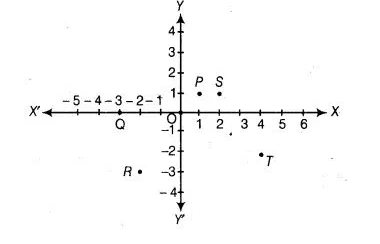# Write the coordinates of each of the points P, Q, R, S, T and 0 from the figure`
Question:

Write the coordinates of each of the points P, Q, R, S, T and 0 from the figureThinking Process

(i) Firstly, draw the perpendicular lines from the point to the coordinates axes.

(ii) Further, measure the distance from intersecting points to the origin along their sign.

(iii) Finally, write the x unit distance and y unit distance in pair.

Solution:

Here, points P and S lie in I quadrant so their both coordinates will be positive. Now, perpendicular distance of P from both axes is 1, so

coordinates of P are (1, 1). Also, perpendicular distance of S from X-axis is 1 and from Y-axis is 2, so coordinates of S are (2,1). Point 0 lies on X-

axis in negative direction so its y-coordinate will be zero and x-coordinate will be -3. So, coordinates of 0 are (-3 0). Point R lies in III quadrant, so

its both coordinates will be negative. Now, its perpendicular distance from X-axis is 3 and from Y-axis is 2, so coordinates of point R are (-2, – 3).

Point T lies in IV quadrant, so its x-coordinate will be positive and y-coordinate will be negative. Now, its. perpendicular distance from X-axis is 2

and from Y-axis is 4, so coordinates of T are (4, -2). Point 0 is the intersection of both axes, so it is the origin and its coordinates are O (0,0).